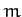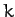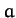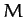Next: Gravitational Potential Theory Up: Coupled Oscillations Previous: Triatomic Molecule

# Exercises

1. A particle of massis attached to a rigid support by means of a spring of spring constant. At equilibrium, the spring hangs vertically downward. An identical oscillator is added to this system, the spring of the former being attached to the mass of the latter. Calculate the characteristic frequencies for one-dimensional vertical oscillations, and describe the associated normal modes.
2. A simple pendulum consists of a bob of masssuspended by an inextensible light string of length. From the bob of this pendulum, a second identical pendulum is suspended. Consider the case of small angle oscillations in the same vertical plane. Calculate the characteristic frequencies, and describe the associated normal modes.
3. A thin hoop of radiusand massoscillates in its own plane (which is constrained to be vertical) hanging from a single fixed point. A small massslides without friction along the hoop. Consider the case of small oscillations. Calculate the characteristic frequencies, and describe the associated normal modes.
4. A simple pendulum of massand lengthis suspended from a block of masswhich is constrained to slide along a frictionless horizontal track. Consider the case of small oscillations. Calculate the characteristic frequencies, and describe the associated normal modes.
5. A thin uniform rod of massand lengthis suspended from one end by a light string of length. The other end of the string is attached to a fixed support. Consider the case of small oscillations in a vertical plane. Calculate the characteristic frequencies, and describe the associated normal modes.
6. A triatomic molecule consists of three atoms of equal mass. Each atom is attached to the other two atoms via identical chemical bonds. The equilibrium state of the molecule is such that the masses are at the vertices of an equilateral triangle of side. Modeling the chemical bonds as springs of spring constant, and only considering motion in the plane of the molecule, find the vibrational frequencies and normal modes of the molecule. Exclude translational and rotational modes.Next: Gravitational Potential Theory Up: Coupled Oscillations Previous: Triatomic Molecule
Richard Fitzpatrick 2011-03-31